8.1

#### Lecture 29: Priority Queues and Heapsort

Introducing the priority queue structure; using a priority queue to conveniently implement a sorting algorithm

##### Motivation

In real life, we often encounter scenarios that involve queuing, such as standing in line at a grocery store or at the movies, or waiting for a haircut. In these “first come, first served” situations, the first person to arrive is the first person served. We can represent this kind of situation with a queue, where we add items to the back of the queue, and remove them from the front.

However, there are other scenarios where the first person to arrive is not the first person served: when boarding an airplane, even if you are the first on line, the first-class passengers will board before you. Or consider patients in an emergency room: if someone arrives early with a comparatively minor injury, and another patient arrives with a more serious problem, the second patient will be treated first. Here, the scenario is more of a “first come, most-important served” situation. How might we represent this data?

##### 29.1Priority Queues

The second scenario above differs from the first because incoming items (patients) are not treated equally: some are more important, or higher-priority, than others. We need to express these priorities somehow in our representation. Armed with our new appreciation for big-O notation and algorithmic efficiency, we want to design a datatype that is tailored to support the following three operations as efficiently as possible:
• Add an item, with a given priority, into the data structure

• Remove the most important item from the data structure

• Get the number of items in the data structure

That’s it — nothing else must be supported. (We may be able to support other items later, but for now, we don’t need to.) This structure is very similar to the queue we discussed before, but has been enhanced with priorities. It is therefore, unsurprisingly, known as a priority queue.

In the rest of this lecture, we are going to make the following simplification: rather than focusing on adding an item paired with a priority, we are going to instead just consider adding a given priority. (We can add the item data as well if we just construct a custom IComparator object that ranks items based on priorities.) Further, we will choose to represent priorities simply as integers, though any type that can be sorted by a comparator will suffice.

Exercise

After completing this lecture, try adapting the data types here to store arbitrary data with priorities, instead of just priorities.

##### 29.2Using lists to implement priority queues: too slow

We might imagine that since a priority queue is “like a queue, but with priorities”, we should be able to implement it using a simple list or Deque<Integer>. We can indeed manage this: we just need to maintain our list in decreasing sorted order. Then the maximum value (i.e., the highest-priority item) will be the first item of the list, and so is readily available in constant (i.e. $$O(1)$$) time. But adding an item to a sorted list is expensive: in the worst case, we constantly add a low-priority item, and so for a priority queue already containing $$n$$ elements, we would have to walk the entire list to find the right place to insert the item, which takes linear (i.e. $$O(n)$$) time.

Do Now!

Would using an ArrayList<Integer> instead of a Deque<Integer> or IList<Integer> be any more efficient?

ArrayLists do not support efficiently inserting an item into the middle of the list (since they have to shove all the subsequent items over by one index), so even though we could use binary search to find the appropriate index at which to insert, the insertion itself is still $$O(n)$$. Conversely, Deques support constant-time insertion (since we just have to fix up four links), but they don’t support binary search, so finding the place to insert costs $$O(n)$$ time.

Can we do better?

##### 29.3Choosing a better data structure for the task

The downside to using a standard linked list is that accessing items near the end of the list is too expensive, so inserting items near the end of the list is too expensive. Conversely, the downside to ArrayLists is that they don’t support inserting items into the middle of the list efficiently. Both data structures efficiently support finding the first item of the list, though. So we can ask the following:

Do Now!

Is there another data structure we have seen where accessing one particular value is extremely efficient (i.e., constant time), and accessing any item is still more efficient than $$O(n)$$?

One compelling possibility is to use a binary tree: accessing the root of the tree takes constant time, and accessing any item in the tree takes $$O(\mathit{depth\ of\ tree})$$. But using an arbitrary binary tree is not enough: technically, the following tree is a binary tree: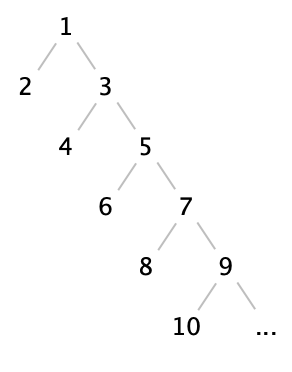Every level of the tree (except the first) contains two items, so this kind of tree of height $$h$$ contains only $$2h$$ items. Said another way, accessing item $$n$$ takes $$n/2$$ steps, or $$O(n)$$ or linear time.

Of course, this is a pathologically bad binary tree — it’s not taking much advantage of the tree shape. Suppose we considered a binary tree that contains as many nodes as possible for its height: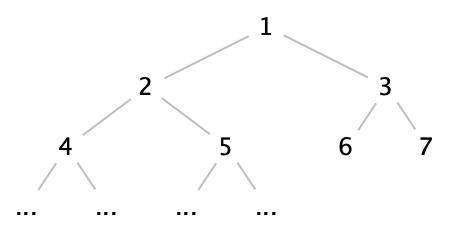Do Now!

For a binary tree of height $$h$$, what is the maximum number of nodes that can be present? Conversely, for a tree with $$n$$ nodes, what is the minimum tree height needed to fit them all?

Since the number of nodes in each level of a binary tree doubles from one level to the next, the maximum number of nodes in a binary tree of height $$h$$ is $$2^h - 1$$. Conversely, a tree of $$n$$ nodes requires at least $$\log_2 n$$ levels to fit them all. This is encouraging: if we somehow enforce that our tree be “as full as possible”, then we ensure that it is as short as possible, too.

In fact, let’s elevate this goal to a definition: a full binary tree is a binary tree where every level (except possibly the last) is completely full, and the last level of the tree is filled from left to right. Accordingly: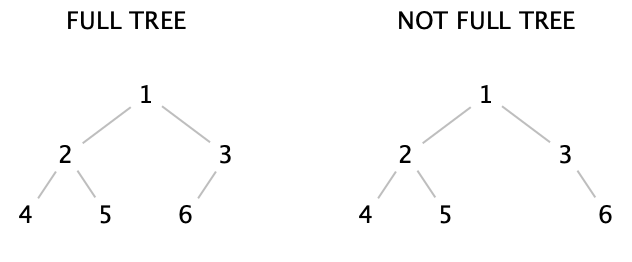We can now successfully access any item in the tree in logarithmic time, and the root of the tree in constant time. Now if only we can ensure that the root of the tree always contains the maximum value, we’d be very nearly done! How can we do that?

##### 29.4Heaps and invariants

Recall for a moment the definition of a binary search tree: it is a binary tree on which we enforce a particular property:
• Every value in the left subtree of a node must be less than or equal to the value at the node,

• Every value in the right subtree of a node must be greater than or equal to the value at the node, and

• Both the left and right subtrees must themselves be valid binary search trees.

This property is called an invariant: we ensure that it is true throughout our program’s usage of the data structure, and can therefore rely on and take advantage of it being true whenever we need to.

What invariants might we want for our priority queue? We want the maximum value to be at the root, certainly, but let’s think ahead: once we remove the maximum value, where should the next-largest value be? It seems reasonable to want the next-largest item be as close to the top as possible. So we codify this idea as the following invariant, known as the heap invariant:
• Every value in the both the left and right subtrees of a node must be less than or equal to the value at the node, and

• Both the left and right subtrees must themselves be valid heaps.

(Notice how similar in spirit the heap invariant is to the binary search tree invariant: it asserts an ordering on the value of a node compared to the values in the subtrees, and asserts the property recursively on all subtrees.) Therefore: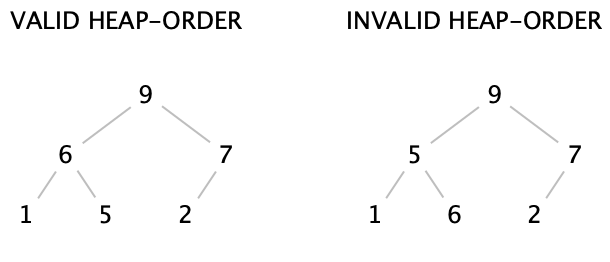In particular, the left tree is a valid heap, even though the values in the lowest level are not sorted — because we’re not trying to sort our data! The right tree is not a valid heap, because 5 has a child 6, which is not in the correct heap order.

We now have two different invariants: a structural one (fullness) that ensures our trees will be conveniently short, and a logical one (heap-ordering) that ensures the values are ordered conveniently for our purposes. How can we exploit this?

##### 29.5Designing a priority queue

Let’s now figure out how to define the operations add and removeMax. (In the next section, we’ll see how to represent this design and implement it conveniently.) We’ll start by asserting that our priority queue will be represented by a heap, that is, a full binary tree that obeys the heap-ordering property. Here is an example of a valid heap:Do Now!

Confirm that this tree is full and that it obeys the heap-ordering property.

##### 29.5.1Adding items to a heap

Let’s try to add 70 to this heap. Where should we add it? We have to ensure that we preserve both invariants, but no matter where we put 70, it looks like we’ll violate at least one of them: if we add it next to the 15, then it violates the heap-ordering property (because it is greater than its parent), and if we add it anywhere else, we violate the fullness property. What to do?

Since adding 70 next to the 15 at least preserves the fullness property, perhaps we can start with that and then “fix up” the tree to restore the heap-ordering invariant.

Do Now!

If we add 70 next to the 15, what should we do to restore the heap-ordering property?

• Insert the 70 in the next available space, to preserve the fullness property: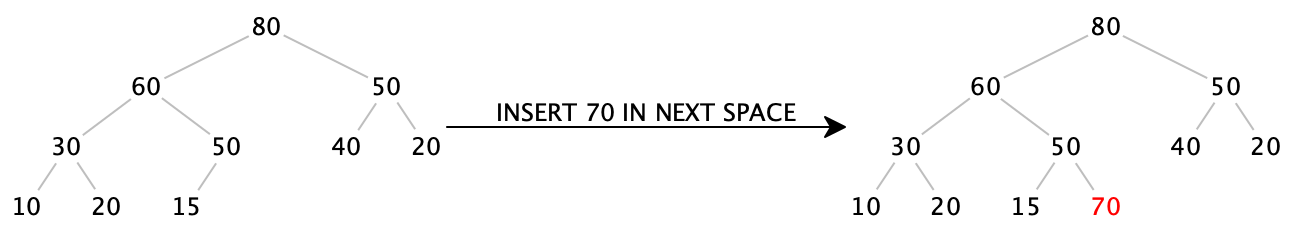• Notice that 70, on its own, is a valid heap. But unfortunately, since 70 is greater than 50, the subtree starting at 50 is not. But we can fix that, if we just swap the two values: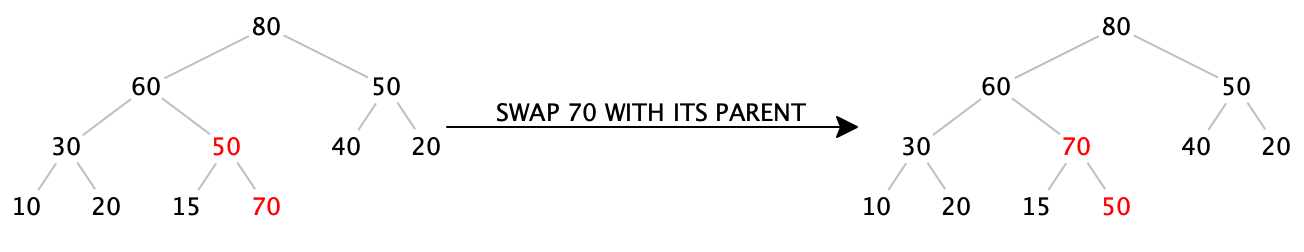Now the subtree starting at 70 is a valid heap. Progress!

• We just ensured that the subtree starting at 70 is a valid heap, but since 70 is greater than 60, the subtree starting at 60 is not. But we can fix that, by swapping again: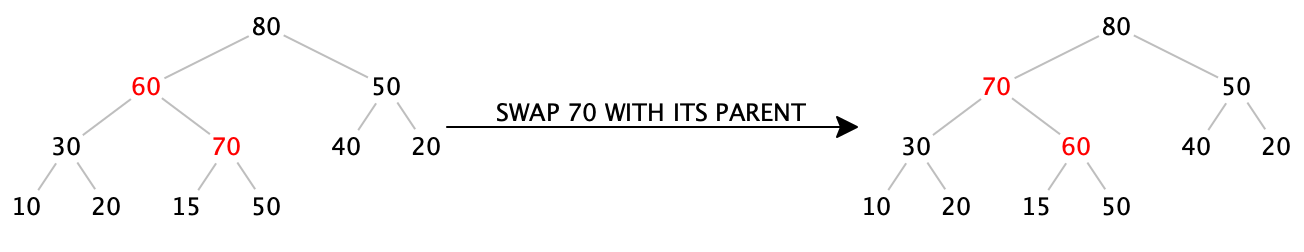Again, the subtree starting at 70 is a valid heap. More progress!

• We just ensured that the subtree starting at 70 is a valid heap. And in fact, since 70 is less than 80, the entire tree is now a valid heap again. Done!

We can codify the procedure above as follows: To add an item into a heap,
• Insert the item in the next available space, maintaining the fullness property.

• While the item is greater than its parent, swap it with its parent. (This step is often known as the “upheap” operation.)

Do Now!

Insert 90 into the final heap above.

• Insert 90 into the next available space: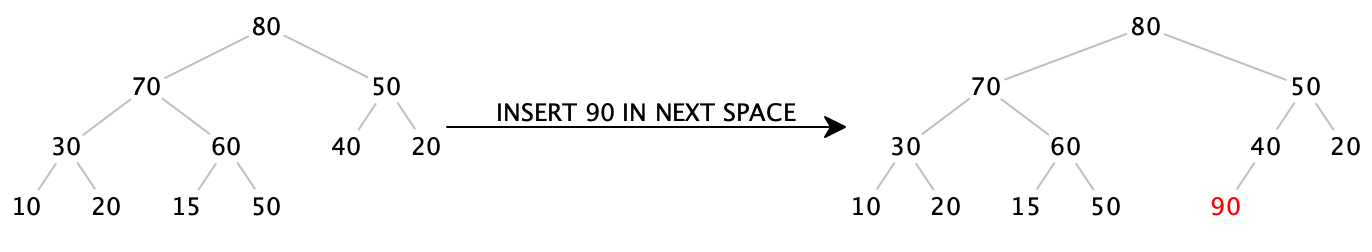• While 90 is greater than its parent, swap it with its parent: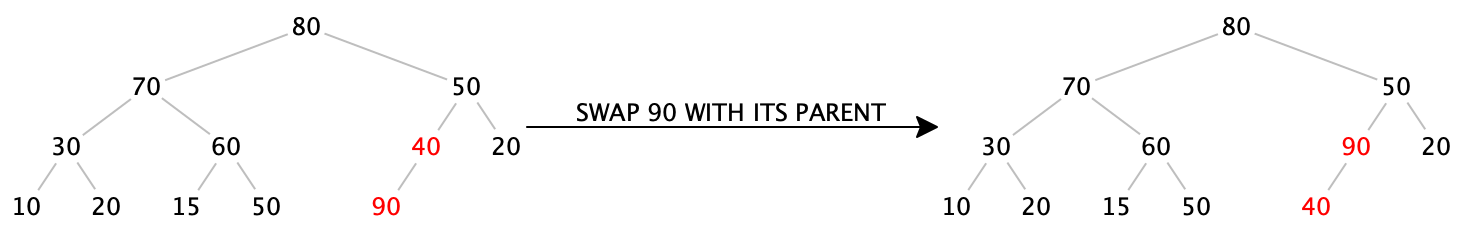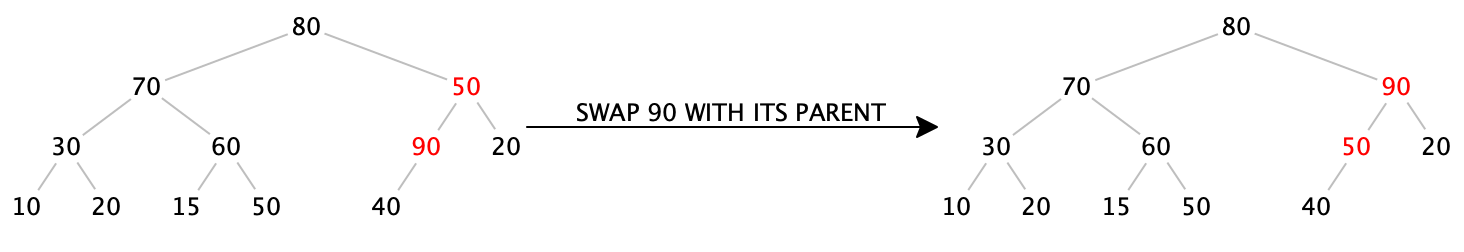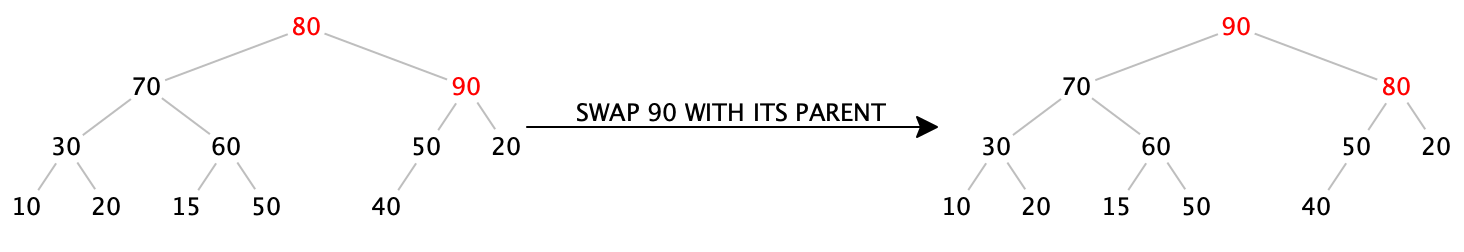We now have a procedure that seems to work to insert an item into a heap. Does it always work, and does it always terminate? We know that it preserves the fullness property, because by construction, we always insert the item into the next available location. And the whole point of swapping the item with its parent is to restore the heap-ordering property. Further, every time we swap the inserted item with its parent, we get closer and closer to the root, so eventually we must reach the root and terminate. At that point, we have preserved the fullness property and restored the heap-ordering property, so this does work in all cases.

Next, how efficient is it? Assuming we can easily (as in, constant-time) find the next available spot to insert the item, the fullness property ensures that our tree has height $$O(\log_2 n)$$, so the upheap operation takes at most $$O(\log_2 n)$$ time. Therefore, our insertion operation happens in logarithmic time — much better than linear. (Of course, there’s a big assumption here, that we can easily find the spot at which to insert...and to see that we can do this efficiently, we’ll need one clever implementation choice, which we’ll address in the next section.)

##### 29.5.2Removing the maximum item from a heap

The maximum item of the heap is easily available: it’s sitting right at the root. If we just blindly “remove” the root, we have a hole in our tree, which breaks the tree in half. How can we fix this? It’s tempting to try to “undo” the upheap operation, but it’s much harder to figure out how to (efficiently) get from the root to the end of the tree than it is to figure out how to get from the end of the tree to the root. (Without examining the entirety of the tree, there’s no way to know whether to swap the root downwards to the right or to the left.)

The key insight in inserting an item was to preserve the fullness property at all costs, and then to fix up the heap-ordering property afterward. Can we do something similar here?
• Remove 90 from the root: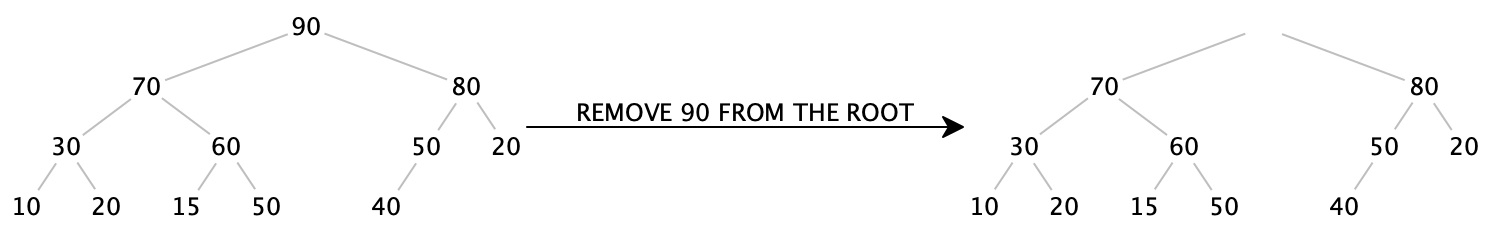• We can try simply moving the last item in the tree up into the root, which will ensure the fullness property stays true, but violates the heap-ordering property: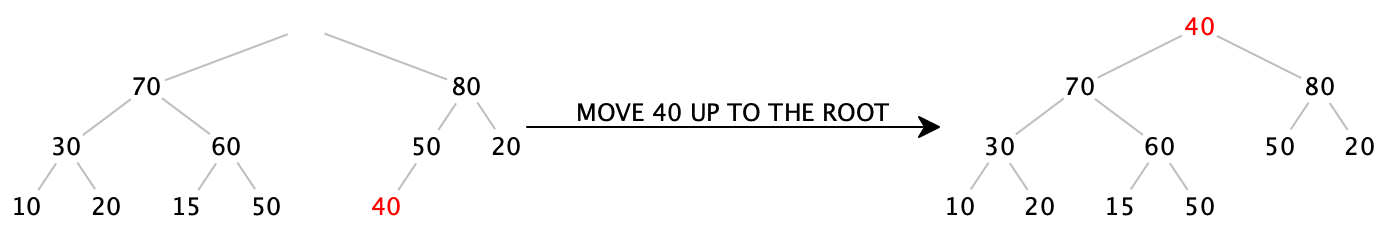• Notice that every node in this tree obeys the heap-ordering property except for the root itself. If we swap the 40 with one of its kids, we can push the problem down a level. We have to choose the larger of the two children, or else we’d create another heap-ordering violation: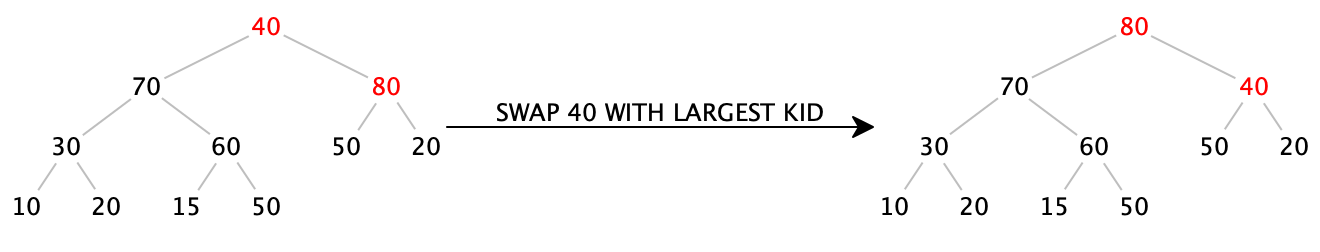• Again, every node in this tree obeys the heap-ordering property except for the node containing 40 itself. So again, we swap it with its largest child: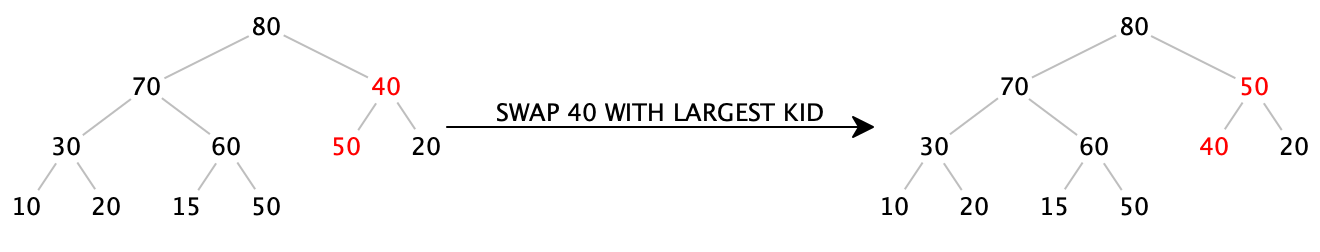• Now 40 is a leaf, so there is no more heap-ordering violation. Done!

We can codify the procedure above as follows: to remove the maximum item,
• Remove the root, and replace it with the last leaf of the tree, maintaining the fullness property.

• While the item is greater than either of its children, swap it with the largest of its children. (This step is often known as the “downheap” operation.)

Do Now!

Remove the next maximum value from the heap above.

• Remove the root, and replace it with the last leaf of the tree: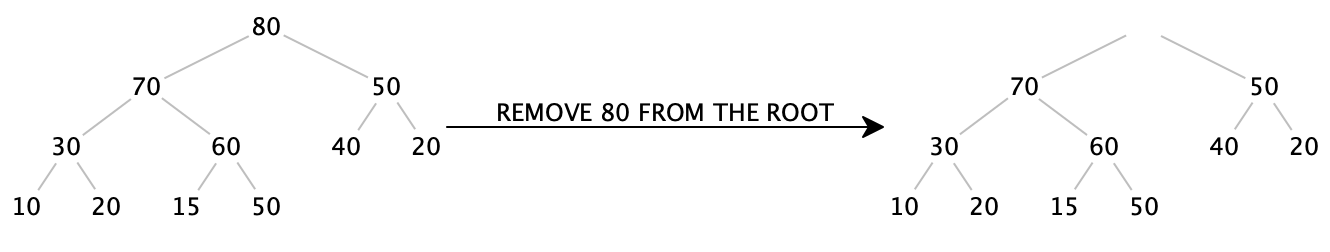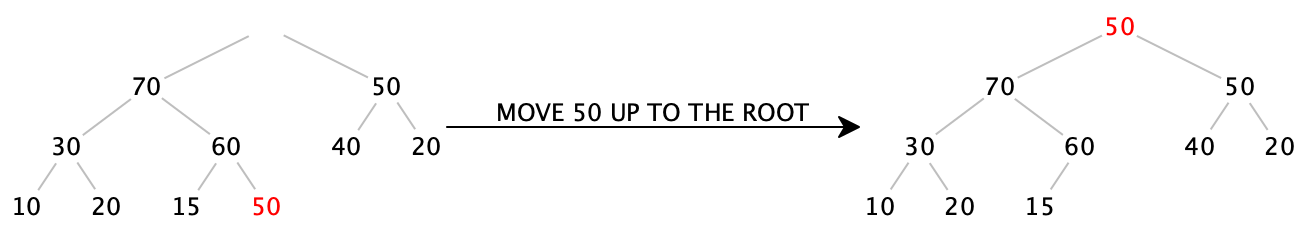• While 50 is greater than either of its children, swap it with the largest of its children.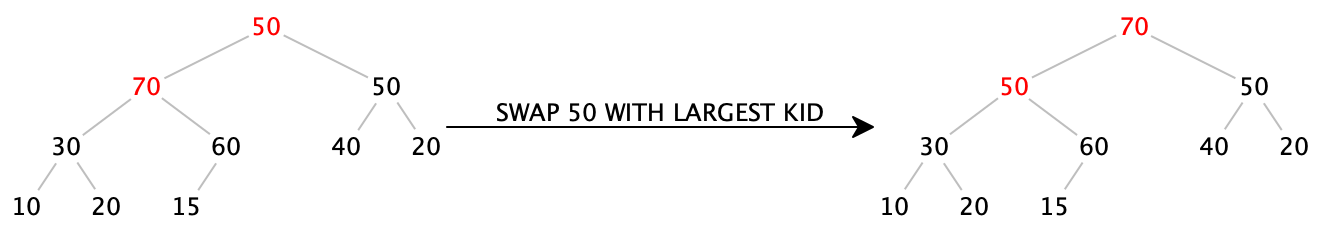Again we have to ask, does this procedure always work, and how efficient is it? Since we replace the root with the last item, we preserve the fullness property by construction. And the whole point of the downheap operation is to restore the heap-ordering property. Since every time we swap the item downward, we come closer to the leaves of the tree, we can only swap a finite number of times before terminating.

Again assuming we can find the last item efficiently, moving it to the root takes constant time, and the downheap operation runs in time proportional to the height of the tree, which, thanks to the fullness property, is logarithmic in the size of tree. Perfect.

##### 29.6Implementing a priority queue

Now we come to the clever implementation trick that makes heaps so efficient to implement. Let’s index each of the nodes in our tree, starting at the root and moving left to right in each level. Here, the indices of each node are shown colored and in brackets: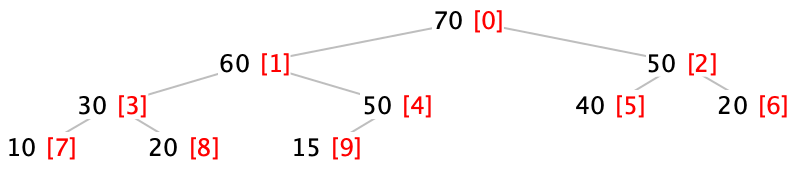Do Now!

Find a formula to compute the index of the left child of a node given the index of a node. Find a similar formula to compute the index of the right child of a node given the index of a node. Finally, find a formula to compute the index of the parent of a node given the index of a node.

Remembering that every level is twice as big as the previous one, and with a little experimentation, we’re led to the following formulas:
• $$leftChild(idx) = 2 * idx + 1$$

• $$rightChild(idx) = 2 * idx + 2$$

• $$parent(idx) = floor((idx - 1) / 2)$$

This is terribly convenient: this means that we don’t have to explicitly store the links between nodes in the tree, if we simply store the items in each node at appropriately numbered indices in some other data structure...

Let’s represent the tree above as an ArrayList<Integer>. The root will be at index 0, and the children of each node will be at their appropriate indices. Now we can see why the fullness property insists that the last layer of the tree be filled from left to right: a full tree containing $$n$$ nodes will simply be stored in indices $$0$$ through $$n-1$$ of an ArrayList, with no gaps! And even better, we now can see that finding the next available location in the tree is trivial: it’s simply the next index in the ArrayList.

So our initial heap example,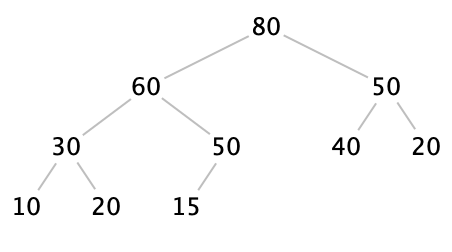can be represented by the ArrayList<Integer>:
Index:   0    1    2    3    4    5    6    7    8    9
Data: [ 80 | 60 | 50 | 30 | 50 | 40 | 20 | 10 | 20 | 15 ]
Let’s recap the two insertions and the two removals that we did on the trees above, using this new representation.

• Inserting 70:
   0    1    2    3    4    5    6    7    8    9
[ 80 | 60 | 50 | 30 | 50 | 40 | 20 | 10 | 20 | 15 ]
===Add 70 to end of ArrayList===>
0    1    2    3    4    5    6    7    8    9   10
[ 80 | 60 | 50 | 30 | 50 | 40 | 20 | 10 | 20 | 15 | 70 ]
===Swap 70 (at index 10) with parent (at index (10-1)/2 = 4)==>
0    1    2    3    4    5    6    7    8    9   10
[ 80 | 60 | 50 | 30 | 70 | 40 | 20 | 10 | 20 | 15 | 50 ]
===Swap 70 (at index 4) with parent (at index (4-1)/2 = 1)===>
0    1    2    3    4    5    6    7    8    9   10
[ 80 | 70 | 50 | 30 | 60 | 40 | 20 | 10 | 20 | 15 | 50 ]

• Inserting 90:
   0    1    2    3    4    5    6    7    8    9   10
[ 80 | 70 | 50 | 30 | 60 | 40 | 20 | 10 | 20 | 15 | 50 ]
===Add 90 to end of ArrayList===>
0    1    2    3    4    5    6    7    8    9   10   11
[ 80 | 70 | 50 | 30 | 60 | 40 | 20 | 10 | 20 | 15 | 50 | 90 ]
===Swap 90 (at index 11) with parent (at index (11-1)/2 = 5)==>
0    1    2    3    4    5    6    7    8    9   10   11
[ 80 | 70 | 50 | 30 | 60 | 90 | 20 | 10 | 20 | 15 | 50 | 40 ]
===Swap 90 (at index 5) with parent (at index (5-1)/2 = 2)===>
0    1    2    3    4    5    6    7    8    9   10   11
[ 80 | 70 | 90 | 30 | 60 | 50 | 20 | 10 | 20 | 15 | 50 | 40 ]
===Swap 90 (at index 2) with parent (at index (2-1)/2 = 0)===>
0    1    2    3    4    5    6    7    8    9   10   11
[ 90 | 70 | 80 | 30 | 60 | 50 | 20 | 10 | 20 | 15 | 50 | 40 ]

Do Now!

Confirm that these operations correspond exactly to the tree diagrams above.

To remove an item, we’ll be a little bit clever: instead of outright removing the root item and replacing it with the last item, we’ll swap them instead. (We might as well, since we need to keep track of the item we’re removing, and we conveniently have space available once we move the last item into the root position!)
• Removing maximum:
   0    1    2    3    4    5    6    7    8    9   10   11
[ 90 | 70 | 80 | 30 | 60 | 50 | 20 | 10 | 20 | 15 | 50 | 40 ]
===Swap root item with last item in heap===>
0    1    2    3    4    5    6    7    8    9   10 ||| 11
[ 40 | 70 | 80 | 30 | 60 | 50 | 20 | 10 | 20 | 15 | 50 ||| 90 ]
===Swap 40 (at index 0) with larger of children (indices 1 and 2)===>
0    1    2    3    4    5    6    7    8    9   10 ||| 11
[ 80 | 70 | 40 | 30 | 60 | 50 | 20 | 10 | 20 | 15 | 50 ||| 90 ]
===Swap 40 (at index 2) with larger of children (indices 5 and 6)===>
0    1    2    3    4    5    6    7    8    9   10 ||| 11
[ 80 | 70 | 50 | 30 | 60 | 40 | 20 | 10 | 20 | 15 | 50 ||| 90 ]
Notice that even though index 5’s left child should be at index 11, we have to remember that we’ve removed 90 from the heap, so index 11 is no longer part of our heap (which we denote with extra vertical bars).

• Removing maximum:
   0    1    2    3    4    5    6    7    8    9   10 ||| 11
[ 80 | 70 | 50 | 30 | 60 | 40 | 20 | 10 | 20 | 15 | 50 ||| 90 ]
===Swap root item with last item in heap===>
0    1    2    3    4    5    6    7    8    9 ||| 10   11
[ 50 | 70 | 50 | 30 | 60 | 40 | 20 | 10 | 20 | 15 ||| 80 | 90 ]
===Swap 50 (at index 0) with larger of children (indices 1 and 2)===>
0    1    2    3    4    5    6    7    8    9 ||| 10   11
[ 70 | 50 | 50 | 30 | 60 | 40 | 20 | 10 | 20 | 15 ||| 80 | 90 ]
===Swap 50 (at index 1) with larger of children (indices 3 and 4)===>
0    1    2    3    4    5    6    7    8    9 ||| 10   11
[ 70 | 60 | 50 | 30 | 50 | 40 | 20 | 10 | 20 | 15 ||| 80 | 90 ]
===Swap 50 (at index 4) with larger of children (index 9)===>
0    1    2    3    4    5    6    7    8    9 ||| 10   11
[ 70 | 60 | 50 | 30 | 50 | 40 | 20 | 10 | 20 | 15 ||| 80 | 90 ]

• Let’s remove the maximum one more time, just for practice:
   0    1    2    3    4    5    6    7    8    9 ||| 10   11
[ 70 | 60 | 50 | 30 | 50 | 40 | 20 | 10 | 20 | 15 ||| 80 | 90 ]
===Swap root item with last item in heap===>
0    1    2    3    4    5    6    7    8 |||  9   10   11
[ 15 | 60 | 50 | 30 | 50 | 40 | 20 | 10 | 20 ||| 70 | 80 | 90 ]
===Swap 15 (at index 0) with larger of children (indices 1 and 2)===>
0    1    2    3    4    5    6    7    8 |||  9   10   11
[ 60 | 15 | 50 | 30 | 50 | 40 | 20 | 10 | 20 ||| 70 | 80 | 90 ]
===Swap 15 (at index 1) with larger of children (indices 3 and 4)===>
0    1    2    3    4    5    6    7    8 |||  9   10   11
[ 60 | 50 | 50 | 30 | 15 | 40 | 20 | 10 | 20 ||| 70 | 80 | 90 ]

Do Now!

What’s accumulating in the tail of the ArrayList, after each removal?

##### 29.7Heapsort

As a convenient side-effect of the removeMax procedure, the tail of our ArrayList accumulates the elements of our list in sorted order. In other words, if we remove every item from our heap, we’ll wind up with a sorted list! This suggests a particularly efficient, and amazingly simple, algorithm for sorting:
 To heapsort a list: Build a heap from all the items in the list. Remove all the items from the heap.
How do we build a heap? Well, we start with an empty heap, and add items into it one by one. Suppose we have an ArrayList of data. We can represent the empty heap as just the first zero items of the ArrayList. We can add the first item of data (at index 0) to the empty heap easily enough — there’s nothing to do. To add the next item of data (at index 1) to the heap, we need to add it to the end of the heap (i.e., put it at index 1, where it already is), and then run upheap on it. In general,
 To build a heap from an ArrayList: For each i from 1 to the size of the ArrayList, upheap(i) To upheap at index idx: Let parentIdx = floor((idx - 1) / 2) If the item at index idx is greater than the item at index parentIdx: Swap items at indices idx and parentIdx upheap(parentIdx) To remove the maximum item: Swap the item at index 0 with the item at the index of the last heap item downheap(0) To downheap at index idx: Let leftIdx = 2 * idx + 1 Let rightIdx = 2 * idx + 2 If the item at index idx is less than either of the items at leftIdx or rightIdx: Let biggestIdx = index of the largest of the items at leftIdx or rightIdx Swap items at indices idx and biggestIdx downheap(biggestIdx)

How efficient is heapsort? Building the heap inserts $$n$$ items, and each insertion costs $$O(\log_2 n)$$ work, so building takes $$O(n\log_2 n)$$. Removing all the items removes $$n$$ items, and each removal costs $$O(\log_2 n)$$ work, so all the removals together take $$O(n\log_2 n)$$ work also...so the entire algorithm takes $$O(n\log_2 n)$$ work. Notice that unlike quicksort, there is no bad worst-case behavior, and unlike merge-sort, there’s no need for any additional storage. Heapsort is an efficient, in-place sorting algorithm.

Exercise

Implement all the algorithms sketched above in Java.

There is another way to build a heap, which in practice is slightly more efficient than the upheap-based solution above:
 To build a heap from an ArrayList: For each i from the (size of the array list - 1) / 2 down to 0, downheap(i)
Why does this work? The last $$n/2$$ items in the ArrayList are all leaves of the tree (why?), and therefore are already valid heaps of size 1 (again, why?). Therefore, if we look at the heaps starting at their parents, the only place the heap-ordering property could be violated is at the parents themselves, and we have downheap to restore the heap-ordering property when the parent is out of order. And since we count from the middle of the list down to the front, by the time we get to the front items of the list, we’ll already have fixed up their children to be valid heaps, so again the only place the heap-ordering property could be violated is at those front items, so again downheap will fix up any problems.

Exercise

Try working through both versions of buildHeap, using the data [1, 2, 3, 4, 5, 6, 7, 8, 9]. Do you wind up with the same heaps? If not, do you nevertheless wind up with two valid heaps?

Exercise

Try finishing the heapsort algorithm on the two heaps you just obtained.

Exercise

Try working through heapsort on the data [9, 8, 7, 6, 5, 4, 3, 2, 1]. (Hint: buildHeap should be particularly easy!)